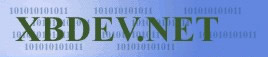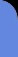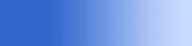Sunday July 12, 2020
 home | about | contact | DonationsSlice of Java. Its simple and its powerful...

If you join two points together, you get a line

by Ben Kenwright

We take two points...p0 and p1...and then we join them together.  Now we will go through the simple process of how to draw a line with java :)  Yup, I'm going to show you the hard way...and the easy way, so you better be prepared and have a good strong cup of coffee at hand, and I would also recommend some of those chocolate biscuits you can dip in as well..hehe.

The tutorials are aimed at 3D, but you'll notice that we are dealing with a 2D screen and simple x and y and no z.  Well your screen is 2D!  Its just pixels...take a close looksy at it, and you'll see lots of dots.  When we render our 3D world on the screen, we'll eventually convert 3D (x,y,z) into 2D (x,y) which we can render.  I'm jumping ahead of myself here...I'll be going into perspective and all sorts of things.  We'll get to all those cool things soon.

 import java.awt.image.*; import java.awt.*; import java.applet.*;   public class line extends Applet {   public void paint(Graphics g)   {            g.drawLine(10,10,200,200);   }// End of paint(..)   }// End of our AppletScreenShot of Applet

What I've showed you here, with this code is how to draw a line the fast way...hehe.  We use this 'drawLine' API a bit more later on as well, when we get into gourand shading...and it allows us to keep a bit of our speed, as the sacrifice of detail.

Lets break this down, and see how we would do this using a simple pixel by pixel method.  We'll not worry to much about optimisation....so we'll just use floats.

We have p0 and p1, each point...has two value...the x and y value.  So we go from x0,y0 to x1,y1.  I always try to start from 0 when I count, I just remember that computers start from 0, so I do as well, and that way I don't get mixed up about if I started at 1 or 0 :)

Difference in x is dx = x1-x0

Difference in y = dy = y1-y0

 Common Errors When you've obtained the dx and dy value, remember that y1 doesn't necessarily have to be greater than y0....the same as x1 being greater than x0.  The two points could be from any two places on the screen.If our dy is positive, it means our line is moving downwards, and the same for dx.  So if dx is negative, it would mean our line is going from p1 to p0.  So be careful if you do loops like this: for(int x=0; x

The secret to drawing lines and many other things with pixels...is interpolation.  As we need to find the x and y position at any point along the line.  Using a simple approach, if we use x as our reference, and x goes from x0 to x1 and we increment it by 1 at a time, we can use our x value to calculate the corresponding y value at that point.  The amount by which y changes for each increase of x is:

yinc = dy/dx;

And the code would be like this:

 Demonstration Code: void drawline( int x0, int y0, int x1, int y1, int colour) {    float dx = x1 - x0;    float dy = y1 - y0;    float yinc = dy/dx;    float y = y0;    for(int x=x0; x

There's a small error in that code which is why you shouldn't run it.  Yup it might work, depending on the points that you use, but if your x1 is less than your x0 value you could have a doozy of a problem on your hands :).

We could fix this problem by using the sign value we get from dx.  And do something like this with the code:

int sx = dx < 0 ? -1 : 1;

And we use sx to increment our x value in the loop, like so:

int xval = x0;

dx = dx * sx; // dx now contains the different between x0 and x1...without any +ve or -ve direction information

for(int x=0; x<dx; x++)

{

setpixel( xval, y, colour)

xval += sx;

y+= yinc;

}

There you have it :)  Thats should work...but wait...there's a further tweek we need to do to make our line drawing function usable.  What if dx is very very tinny...only 1...and dy is long...long...100 pixels for example...now only one pixel will get plotted because where using dx as the interpolation value.

Hence we need to check for the largest difference value...either dx or dy...and determine if we use x or y as the increment by 1, and the other as the increment by a fraction.

So we put the following code together:

 Code Applet: import java.awt.image.*; import java.awt.*; import java.applet.*;   public class line extends Applet {   public void paint(Graphics g)   {            g.setColor( Color.blue );    // We draw a line next to the line using our                g.drawLine(10,11,200,201);  //  line algorithm using java api                drawLine(g,10,10,200,200, Color.green); // Here we use our own line drawing function :)   }// End of paint(..)     void drawLine( Graphics g, float x0, float y0, float x1, float y1, Color colour)   {           float dx = x1 - x0;               float dy = y1 - y0;                 int sx = dx > 0 ? 1 : -1;               int sy = dy > 0 ? 1 : -1;                             dx = dx*sx;               dy = dy*sy;                             float dxdy = dx/dy;               float dydx = dy/dx;                             dxdy *= sx;               dydx *= sy;                             float py = y0;               float px = x0;                                if( dx > dy )               {                                   for(int x=0; x

 Possible Bug - Crash Looking at the code it looks okay, and it does.  You could draw a large number of lines and it would all work fine.  As long as the points are on the screen!  We've not mentioned a situation where we might plot two points that are off screen...or a line that is partly on the screen.  Of course our algorithm at this stage will still work as we are using 'fillRect(..)' as the pixel plotting function, and this function checks for off screen values.  But if we decide to use an array of integers for example we might want to include some error checking incase our applet suddently crashes without notice.

I always think, that when you write a new function....even the simplest one, that you should work a method out to test it to its fullest.  The most effective way to test a function, is usually with a slower more accurate one...and compare the results they generate.. this is how Microsoft tests there Office Excel.  They have one version of the algorithm in asm which is lightening fast...and a slower version in C which is used to test the asm version.

For our little test app, I'll use the mouse and buttons...so if you left click that selects the left point...and if you right click that selects the right point :)  And it will draw the line from the two points...so we can test all sorts of combinations.

I've put together a simple test code below, which plots the a line using the Java *awt method and also with our own line drawing function - and we can compare the lines.  So by clicking the mouse around the window we can select a variety of conditions for our line.

 Testing Line Code: (Download Source Code) /*********************************************************************************************/ /*                                                                                           */ /*  Java 3D Engine Basics Tutorials - Tut Line Drawing                                       */ /*  Auth: bkenwright@xbdev.net                                                               */ /*                                                                                           */ /*  Setting pixels and drawing lines with java 1.1 and later!                                */ /*                                                                                           */ /*********************************************************************************************/   import java.awt.image.*; import java.awt.*; import java.applet.*;   public class line extends Applet {   public void paint(Graphics g)   {            g.drawLine(mouse_x_right,  mouse_y_right,                               mouse_x_left,mouse_y_left);                    drawLine(g,  mouse_x_right,mouse_y_right,                                                        mouse_x_left, mouse_y_left, Color.green);   }// End of paint(..)     void drawLine( Graphics g, float x0, float y0, float x1, float y1, Color colour)   {           float dx = x1 - x0;               float dy = y1 - y0;                 int sx = dx > 0 ? 1 : -1;               int sy = dy > 0 ? 1 : -1;                             dx = dx*sx;               dy = dy*sy;                             float dxdy = dx/dy;               float dydx = dy/dx;                             dxdy *= sx;               dydx *= sy;                             float py = y0;               float px = x0;                                if( dx > dy )               {                                    for(int x=0; x

Well I've tested it out, and it works okay.  But a few words on optimisation.  We really don't want to convert a float to an int all the time in our main loop...I've done it for the x and y...but with optimisation you could do it with only one of the variables.  Or you could take it further and apply the 'Bresenham' algorithm, which would draw a line using only integers.  There always so much more to learn :)

 Visitor: 9534626  { 209.237.238.175 } Copyright (c) 2002-2020 xbdev.net - All rights reserved. Designated tutorial and software are the property of their respective owners.